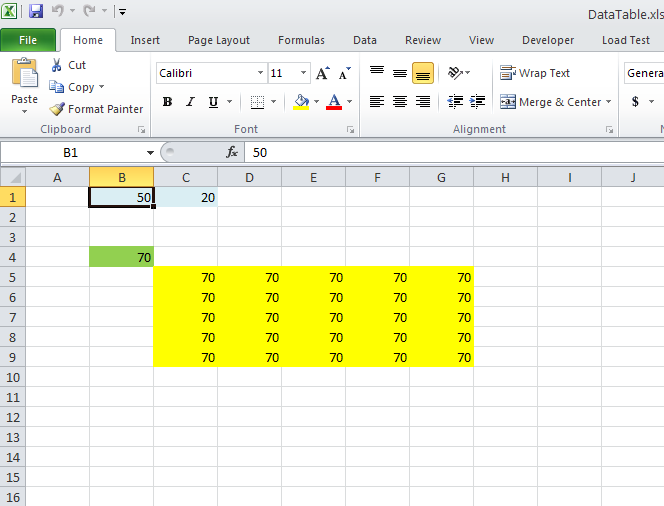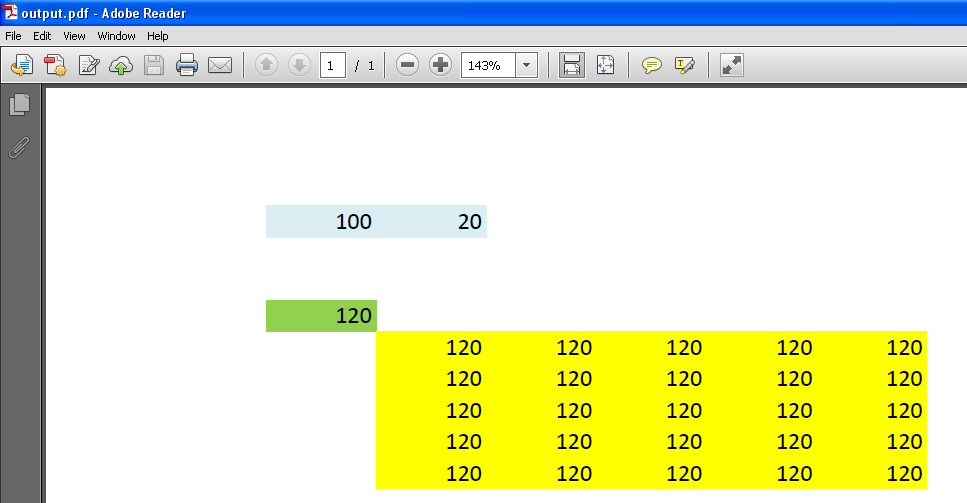# Calculation of Array Formula of Data Tables

Contents
[ ]

## Calculation of Array Formula of Data Tables

In the following sample code, we used this source excel file which is also shown in the following image.If you change the value of cell B1 to 100, the values of Data Table which are filled with Yellow color will become 120. The sample code generates the output PDF which shows 120 as values in the Data Table as shown in this image.Here is the sample code used to generate the output PDF from the source excel file. Please read the comments for more information.